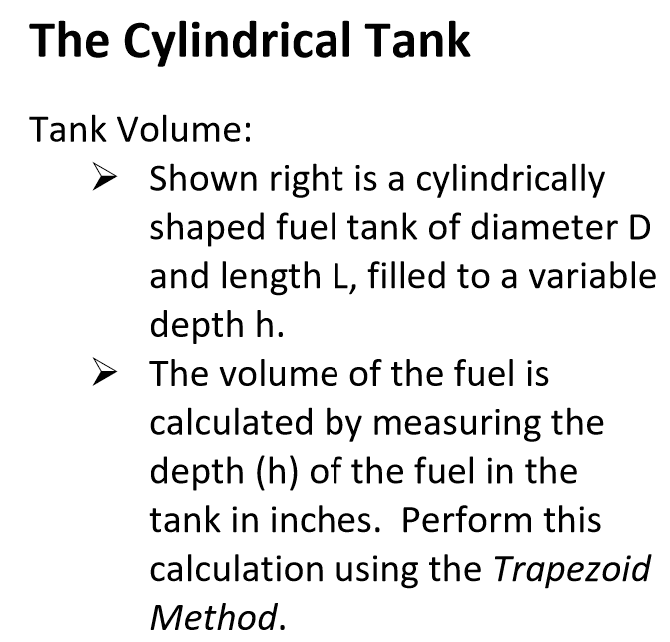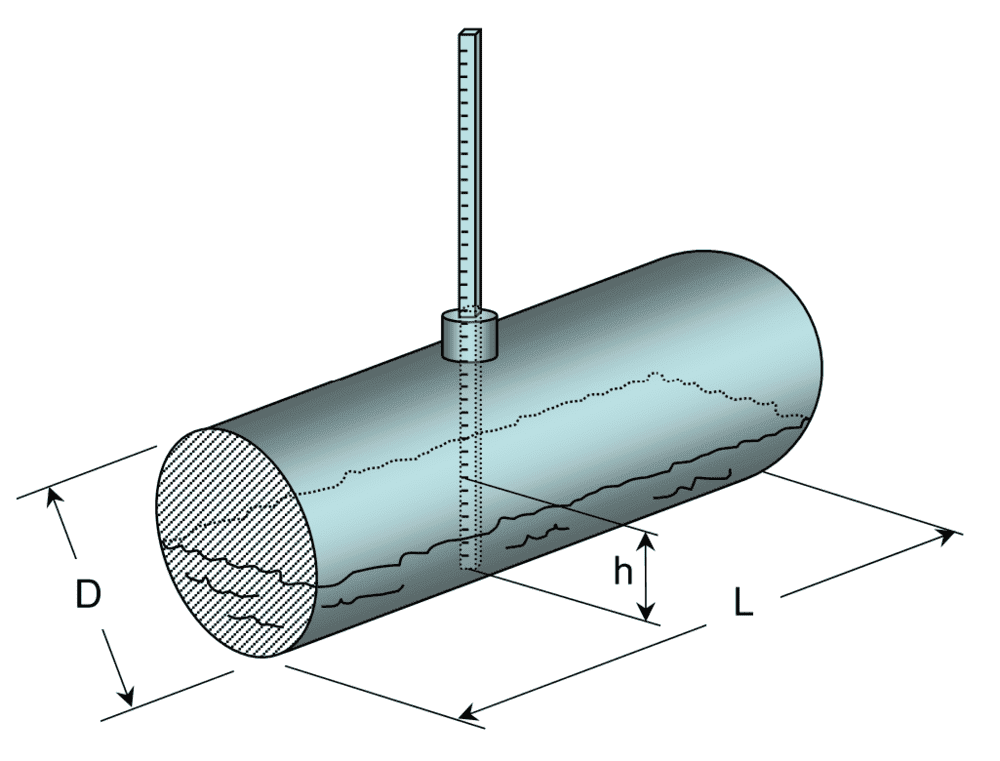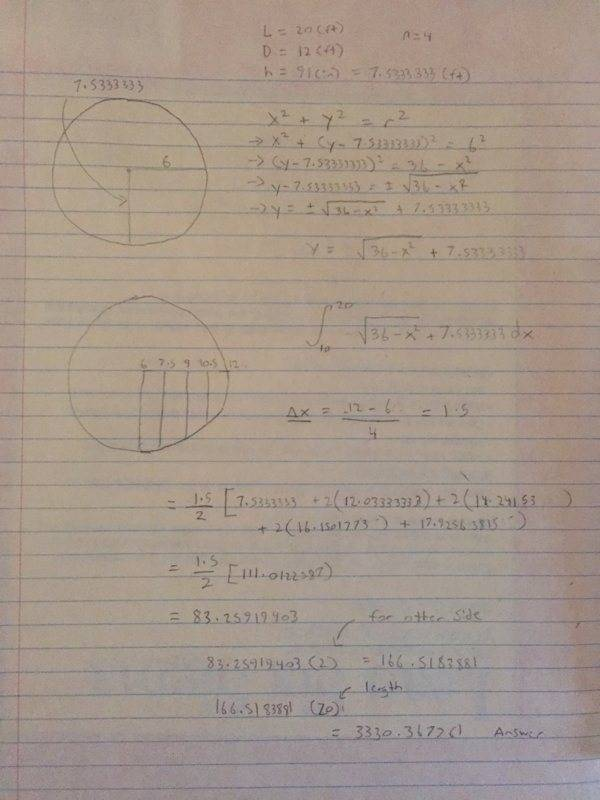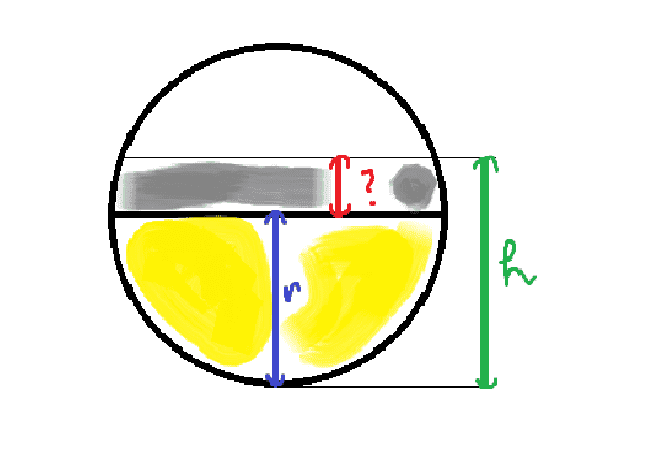# Need help solving this trapezoidal problem

Trey Chandler
Homework Statement:
Shown below is a cylindrically shaped fuel tank of diameter D and length L, filled to a variable depth h. The volume of the fuel is calculated by measuring the depth (h) of the fuel in the tank in inches. Perform this calculation using the Trapezoid Method. Plug in the values given to you (in a separate file) to find the volume, measured in gallons.

My values are D = 12(ft), L = 20(ft), h = 91(in)
Relevant Equations:
x^2 + y^2 = r2This is my attempt at the problem I'm pretty sure the answer is supposed to be in gallons but I don't know what I'm doing clearly.Mentor
It looks like your answer is in units of cubic inches. One gallon = 231 cu. inches.